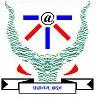Chandler , Phoebe and the Game
Tag(s):

Problem
Editorial
Analytics

## Chandler , Phoebe and the GameChandler has bought a new computer and is now very excited. Seeing his excitement Phoebe asks him about what he is going to do with his new computer. In a rather sarcastic tone, which is rather common for Chandler, he replies for 'Playing games'.

Now Phoebe takes his laptop and gives him a task. Chandler is really eager to play games and therefore asks for your help in solving the problem. The problem is as follows. Pheobe gives Chandler a series of digits (A[i]). He has to report the value of Collision.

Collision is calculated in the following way. Take a prefix of the series and get its Modulus by 1000007. The value you get is a box number X. Now you put that prefix in the box number X. Repeat this process for all prefixes. Phoebe will then ask Q queries from Chandler. Each query will be a box number X. You have to tell the count of prefix in these boxes.

Input:

T - denoting the number of test cases
in every test case
N - denoting the number of digits in series
Then N digits seperated by spaces.
Q - denoting the number of queries
then Q lines follow each containing a box number B[i]

Output:

On every line the count of prefix in that box.

Constraints:

$1 \leq T \leq 10$

$1 \leq N , Q \leq 10^{5}$

$0 \leq A[i] \leq 9$

$0 \leq X \leq 1000006$

Problem Setter - Satyarth

SAMPLE INPUT
1
5
1 2 3 4 5
5
1
12
123
124
4
SAMPLE OUTPUT
1
1
1
0
0
Explanation

The series is 1 2 3 4 5

The numbers are 1 % 1000007 = 1
12 % 1000007 = 12
123 % 1000007 = 123
1234 % 1000007 = 1234
12345 % 1000007 = 12345

therefore box 1 , 12, 123, 1234, and 12345 each have one prefix

Time Limit: 2.0 sec(s) for each input file.
Memory Limit: 256 MB
Source Limit: 1024 KB
Marking Scheme: Marks are awarded when all the testcases pass.
Allowed Languages: Bash, C, C++, C++14, Clojure, C#, D, Erlang, F#, Go, Groovy, Haskell, Java, Java 8, JavaScript(Rhino), JavaScript(Node.js), Julia, Kotlin, Lisp, Lisp (SBCL), Lua, Objective-C, OCaml, Octave, Pascal, Perl, PHP, Python, Python 3, R(RScript), Racket, Ruby, Rust, Scala, Swift, Swift-4.1, TypeScript, Visual Basic

## CODE EDITOR

Initializing Code Editor...
Your Rating:

## This Problem was Asked inChallenge Name

Trihacker - IIITA (Mirror)

OTHER PROBLEMS OF THIS CHALLENGE
• Math > Combinatorics
Notifications

?